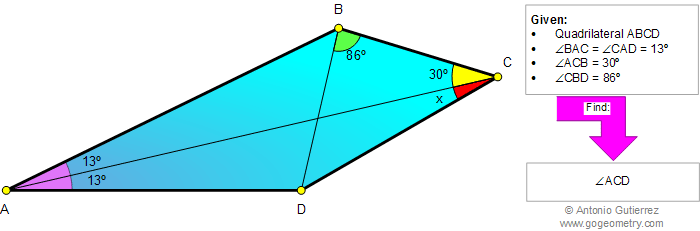# Online Geometry Problem 855: Quadrilateral, Triangle, Angles, 30 degrees, Congruence, Auxiliary lines. Level: High School, SAT Prep, College, Mathematics Education

 The figure below shows a quadrilateral ABCD with angle BAC = angle CAD = 13 degrees, angle ACB = 30 degrees, and angle CBD = 86 degrees. Find the measure of angle ACD. Try to use elementary geometry (no trigonometry).Home | Search | Geometry | Problems | All Problems | Open Problems | Visual Index | 10 Problems | 851-860 | Triangles | Quadrilaterals | Angles | 30 degrees | Email | Solution / comment | By Antonio Gutierrez